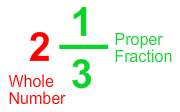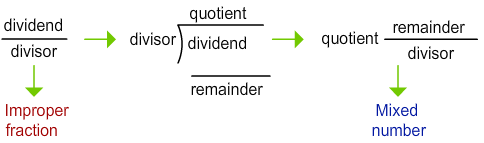# Mixed Numbers

Rate 0 stars Common Core
Quiz size:
Message preview:
Someone you know has shared Mixed Numbers quiz with you:

To play this quiz, click on the link below:

https://www.turtlediary.com/quiz/mixed-numbers.html

Hope you have a good experience with this site and recommend to your friends too.

Login to rate activities and track progress.
Login to rate activities and track progress.

Mixed number is a combination of a whole number and a proper fraction.

For example:To convert an improper fraction into a mixed number, divide the numerator by the denominator.

The quotient becomes the whole number, the remainder becomes the numerator, and the denominator remains the same.To convert a mixed number into an improper fraction:

Multiply the fraction's denominator by the whole number part, and add the product to the numerator. This becomes the numerator of the improper fraction.

The denominator remains the same.ds

A B C D E F G H I J K L M N O P Q R S T U V W X Y Z